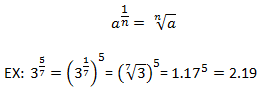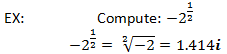# Exponent Calculator

Enter values into any two of the input fields to solve for the third.

## Result

1.000131536000 = INF

Steps:
 1.000131536000 = 1.0001 × 1.0001 × ... × 1.0001 = INF

 ^ = use e as baseRelatedScientific Calculator | Log Calculator | Root Calculator

### What is an exponent?

Exponentiation is a mathematical operation, written as an, involving the base a and an exponent n. In the case where n is a positive integer, exponentiation corresponds to repeated multiplication of the base, n times.

an = a × a × ... × a
n times

The calculator above accepts negative bases, but does not compute imaginary numbers. It also does not accept fractions, but can be used to compute fractional exponents, as long as the exponents are input in their decimal form.

### Basic exponent laws and rules

When exponents that share the same base are multiplied, the exponents are added.

an × am = a(n+m)
EX: 22 × 24 = 4 × 16 = 64
22 × 24 = 2(2 + 4) = 26 = 64

When an exponent is negative, the negative sign is removed by reciprocating the base and raising it to the positive exponent.

a(-n) =
 1 an
EX: 2(-3) = 1 ÷ 2 ÷ 2 ÷ 2  =
 1 8
EX: 2(-3) =
 1 23
=
 1 8

When exponents that share the same base are divided, the exponents are subtracted.

 am an
= a(m - n)
EX:
 22 24
=
 4 16
=
 1 4

 22 24
= 2(2-4) = 2-2
 1 22
=
 1 4

When exponents are raised to another exponent, the exponents are multiplied.

(am)n = a(m × n)
EX: (22)4 = 44 = 256
(22)4 = 2(2 × 4) = 28 = 256

When multiplied bases are raised to an exponent, the exponent is distributed to both bases.

(a × b)n = an × bn
EX: (2 × 4)2 = 82 = 64
(2 × 4)2 = 22 × 42 = 4 × 16 = 64

Similarly, when divided bases are raised to an exponent, the exponent is distributed to both bases.

(
 a b
)n  =
 an bn
EX: (
 2 5
)2  =
 2 5
×
 2 5
=
 4 25
(
 2 5
)2  =
 22 52
=
 4 25

When an exponent is 1, the base remains the same.

a1 = a

When an exponent is 0, the result of the exponentiation of any base will always be 1, although some debate surrounds 00 being 1 or undefined. For many applications, defining 00 as 1 is convenient.

a0 = 1

Shown below is an example of an argument for a0=1 using one of the previously mentioned exponent laws.

If an × am = a(n+m)
Then an × a0 = a(n+0) = an

Thus, the only way for an to remain unchanged by multiplication, and this exponent law to remain true, is for a0 to be 1.

When an exponent is a fraction where the numerator is 1, the nth root of the base is taken. Shown below is an example with a fractional exponent where the numerator is not 1. It uses both the rule displayed, as well as the rule for multiplying exponents with like bases discussed above. Note that the calculator can calculate fractional exponents, but they must be entered into the calculator in decimal form.It is also possible to compute exponents with negative bases. They follow much the same rules as exponents with positive bases. Exponents with negative bases raised to positive integers are equal to their positive counterparts in magnitude, but vary based on sign. If the exponent is an even, positive integer, the values will be equal regardless of a positive or negative base. If the exponent is an odd, positive integer, the result will again have the same magnitude, but will be negative. While the rules for fractional exponents with negative bases are the same, they involve the use of imaginary numbers since it is not possible to take any root of a negative number. An example is provided below for reference, but please note that the calculator provided cannot compute imaginary numbers, and any inputs that result in an imaginary number will return the result "NAN," signifying "not a number." The numerical solution is essentially the same as the case with a positive base, except that the number must be denoted as imaginary.